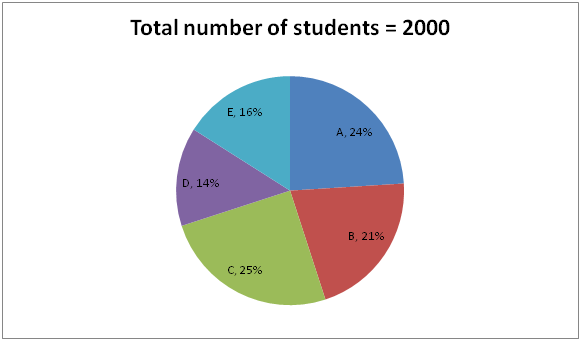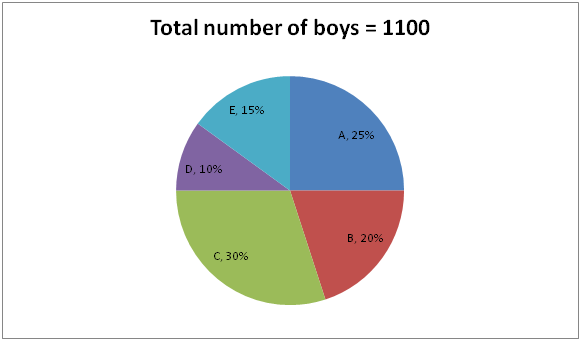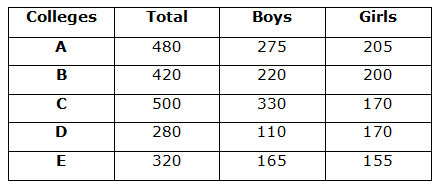# SBI Clerk Pre Quantitative Aptitude (Day-50)

Dear Aspirants, Our IBPS Guide team is providing new series of Quantitative Aptitude Questions for SBI Clerk Prelims 2020 so the aspirants can practice it on a daily basis. These questions are framed by our skilled experts after understanding your needs thoroughly. Aspirants can practice these new series questions daily to familiarize with the exact exam pattern and make your preparation effective.

Start Quiz

Ensure Your Ability Before the Exam – Take SBI Clerk 2020 Prelims Free Mock Test

Data Interpretation

Directions (1 – 5): Study the following information carefully and answer the questions given below?

The first pie chart shows the total number of students in five different colleges and the second pie chart shows the number of boys in different colleges.1) What is the difference between the number of girls in A and E?

a) 20

b) 40

c) 50

d) 60

e) None of these

2) Which of the following colleges has the same number of girl students?

a) A and C

b) C and D

c) E and A

d) B and E

e) None of these

3) The number of boys in D is approximately what percent of the total number of students in B?

a) 24%

b) 26%

c) 28%

d) 30%

e) 32%

4) What is the difference between the average number of boys and girls in all the colleges together?

a) 30

b) 20

c) 50

d) 60

e) None of these

5) The number of boys from B is approximately what percent of the number of boys in C?

a) 33.33%

b) 44.44%

c) 55.56%

d) 66.67%

e) 77.78%

Directions (Q. 6 – 10): In the following questions, two equations I and II are given. You have to solve both the equations and give Answer as,

a) If x > y

b) If x ≥ y

c) If x < y

d) If x ≤ y

e) If x = y or the relation cannot be established

6) I) x2 – x – 56 = 0

II) y2 – 17y + 70 = 0

7) I) 4x2 + 36x + 77 = 0

II) 3y2 + 39y + 126 = 0

8) I) 2x2 – 13x – 57 = 0

II) 3y2 – 14y – 69 = 0

9) I) 3x – 4y = 13

II) 2x – 3y = 11

10) I) x2 – 18x – 243 = 0

II) y2 + 23y + 132 = 0

Directions (1-5) :Required difference = 205 – 155 = 50

College C and D has equal number of girl students.

Required percentage = 110/420 * 100 = 26%

Average number of boys = 1100/5 = 220

Average number of girls = (2000 – 1100)/5 = 900/5 = 180

Difference = 220 – 180 = 40

Required percentage = 220/330 * 100 = 66.67%

Directions (6-10) :

I) x2 – x – 56 = 0

(x + 7) (x – 8) = 0

x = -7, 8

II) y2 – 17y + 70 = 0

(y – 7) (y – 10) = 0

y = 7, 10

Relationship between x and y cannot be established.

I) 4x2 + 36x + 77 = 0

4x2 + 22x + 14x + 77 = 0

2x (2x + 11) + 7 (2x + 11) = 0

(2x + 7) (2x + 11) = 0

x = -3.5, -5.5

II) 3y2 + 39y + 126 = 0

3y2 + 18y + 21y + 126 = 0

3y (y + 6) + 21 (y + 6) = 0

(3y + 21) (y + 6) = 0

y = -7, -6

x > y

I) 2x2 – 11x – 57 = 0

2x2 + 6x – 19x – 57 = 0

2x (x + 3) – 19 (x + 3) = 0

(2x – 19) (x + 3) = 0

x = 9.5, -3

II) 3y2 – 14y – 69 = 0

3y2 + 9y – 23y – 69 = 0

3y (y + 3) – 23 (y + 3) = 0

(3y – 23) (y + 3) = 0

y = 23/3, -3

y = 7.66, -3

Can’t be determined

3x – 4y = 13 –> (1)

2x – 3y = 11 –> (2)

By solving the equation (1) and (2), we get,

x = -5, y = -7

x > y

I) x2 – 18x – 243 = 0

(x – 27) (x + 9) = 0

x = 27, -9

II) y2 + 23y + 132 = 0

(y + 11) (y + 12) = 0

y = -11, -12

x > y

 Check Here to View SBI Clerk Prelims 2020 Quantitative Aptitude Questions Day 49 Day 48 Day 47 Click Here for SBI Clerk 2020 – Detailed Exam Notification